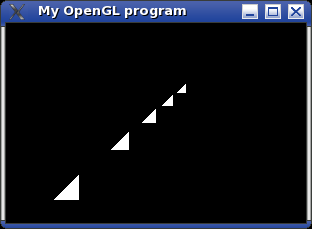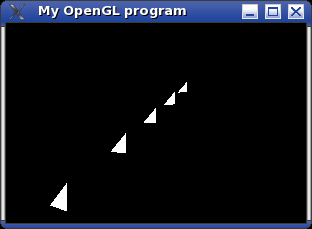# OpenGL Diary

## Day 3 - Placing things into the world

On the previos day, I placed a few triangles at different positions into the "world" by adding values to each of the corners of a "standard triangle".

void draw() {

glClear(GL_COLOR_BUFFER_BIT | GL_DEPTH_BUFFER_BIT);

glBegin(GL_TRIANGLES);

for (float i = 0.0f; i < 1.5f; i += 0.3f) {

glVertex3f(-0.6f + i, -0.6f + i, -1.3f - i * 2);

glVertex3f(-0.4f + i, -0.6f + i, -1.3f - i * 2);

glVertex3f(-0.4f + i, -0.4f + i, -1.3f - i * 2);

}

glEnd();

glFlush();

glutSwapBuffers();

}

This works fine if you only want to move (translate) an object with a small amount of vertices. If the object gets more complex or you want to rotate the object, this is not the best way to do it.

The best way to draw very complex objects in OpenGL is using "display lists". First you create such a list.

void makeTriangle() {

glNewList(1, GL_COMPILE);

glBegin(GL_TRIANGLES);

glVertex3f(-0.2f, 0.0f, 0.0f);

glVertex3f( 0.0f, 0.0f, 0.0f);

glVertex3f( 0.0f, 0.2f, 0.0f);

glEnd();

glEndList();

}

Common trap:

Always remember to call up all functions which create display lists. OpenGL won't complain if you try to draw an empty display list and will simply draw nothing.

I have often spent a lot of time looking for an error elsewhere, when I actually only forgot to call up one of my "makeSomething" functions.

Afterwards we can simply call the display list up, to draw it. Keep in mind that the following code doesn't produce any visible output, since the triangle is clipped because it is too near (z = 0.0).

void draw() {

glClear(GL_COLOR_BUFFER_BIT | GL_DEPTH_BUFFER_BIT);

glCallList(1);

glFlush();

glutSwapBuffers();

}

The triangle can now be drawn at different locations by modifying the modelview matrix. So the matrix mode has to be set to GL_MODELVIEW via the glMatrixMode function. However this is the default mode and we made sure we always return to this mode after manipulating the projection matrix. The modelview matrix can be changed with glTranslate to move the triangle drawing location.

void draw() {

glClear(GL_COLOR_BUFFER_BIT | GL_DEPTH_BUFFER_BIT);

for (float i = 0.0f; i < 1.5f; i += 0.3f) {

glTranslatef(-0.6f + i, -0.6f + i, -1.3f - i * 2);

glCallList(1);

}

glFlush();

glutSwapBuffers();

}

This produces the same picture as the original program.The same result can be obtained with a slightly different approach.

void draw() {

glClear(GL_COLOR_BUFFER_BIT | GL_DEPTH_BUFFER_BIT);

glTranslatef(-0.9f , -0.9f, -0.7f);

for (int i = 0; i < 5; ++i) {

glTranslatef(0.3f, 0.3f, -0.6f);

glCallList(1);

}

glFlush();

glutSwapBuffers();

}

Common trap:

Most of the time you should remember to call glLoadIdentity at the start of the function which does all the drawing, otherwise all the operations you performed on the modelview matrix are still in effect and further operations will not start from a "fresh" matrix.

The other to functions needed to move things around are glRotate and glScale. However some care has to be taken when you combine these with glTranslate.

void draw() {

glClear(GL_COLOR_BUFFER_BIT | GL_DEPTH_BUFFER_BIT);

for (float i = 0.0f; i < 1.5f; i += 0.3f) {

glTranslatef(-0.6f + i, -0.6f + i, -1.3f - i * 2);

glRotatef(-30.0f, 0.0f, 1.0f, 0.0f);

glCallList(1);

}

glFlush();

glutSwapBuffers();

}

This rotates all triangles 30 degrees clockwise around the y-axis (this is because in mathematics positive angles are always measured counterclockwise), giving the following picture.If you call the functions the other way around, you get a different result.

void draw() {

glClear(GL_COLOR_BUFFER_BIT | GL_DEPTH_BUFFER_BIT);

for (float i = 0.0f; i < 1.5f; i += 0.3f) {

glRotatef(-30.0f, 0.0f, 1.0f, 0.0f);

glTranslatef(-0.6f + i, -0.6f + i, -1.3f - i * 2);

glCallList(1);

}

glFlush();

glutSwapBuffers();

}Once you call glRotate (or glScale) the changes apply to all following calls of OpenGL functions. So in the above example the vector passed to glTranslate is first rotated which causes all triangles to appear at a different position.

On Day 4 - What is the matrix? we will take a detailed look at how OpenGL uses the different matrices (e.g. the modelview matrix). Also OpenGL matrix stacks are explained.